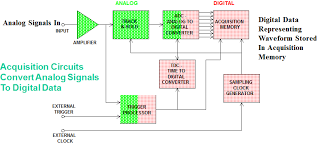## You need to test, we're here to help.

You need to test, we're here to help.

## 01 April 2013

### Oscilloscope Basics: Oscilloscope Bandwidth

Among the most important basic specifications of a digital oscilloscope is its bandwidth. Knowing a bit about bandwidth and the influences on the specification can be very helpful in selecting the right oscilloscope for your application. This post will cover some fundamental aspects of oscilloscope bandwidth.Figure 1: The acquisition circuitry of a digital oscilloscope
When we speak of the bandwidth of an oscilloscope, it is important that we distinguish between analog bandwidth and digital bandwidth. Analog bandwidth is influenced primarily by the instrument's analog input circuits, including the amplifier, track and hold, and analog-to-digital converter (ADC). In the figure at right, we see a generalized block diagram of the waveform-acquisition circuits in a digital oscilloscope. The input amplifier scales the input signal's amplitude to match the input range of the ADC. The track and hold samples the input data at a sampling frequency set by the timebase. The ADC converts the voltage of each sample into a digital value. The resulting digitized samples are stored in acquisition memory until needed for further processing. Generally speaking, the input amplifier is the first bandwidth-deciding element.

Digital bandwidth is largely a function of the instrument's sampling rate. While the analog bandwidth determines the highest frequency that can be fed into the oscilloscope without incurring losses, the digital bandwidth must be high enough to sample that input signal without errors caused by exceeding the Nyquist frequency

The Nyquist limit says that analog signals sampled at greater than two times the highest frequency contained within the signal can be reconstructed with no loss of information. Thus, the highest frequency (digital bandwidth) that can be present in a digitized waveform is one half the sample rate. More practical limits are 1/3 to 1/10th of the sampling rate. Narrowband waveforms such as sine waves can be sampled at a lower rate; broadband signals such as pulses need to be sampled at higher  rates. The consequences of sampling at too low a rate include aliasing and distortion.Figure 2: The frequency at which a sinusoid is attenuated to 70.7% of the initial amplitude is known as the -3 dB point.
Generally speaking, bandwidth is understood as the frequency range over which a given oscilloscope will take accurate measurements and is usually expressed in megahertz (MHz) or gigahertz (GHz). To go a little deeper on the definition, an oscilloscope's bandwidth is the frequency at which a sinusoidal waveform at the instrument's input is attenuated to 70.7% of the initial amplitude on the display. This is known as the –3 dB point (Figure 2).

So how much bandwidth should your oscilloscope have? For one thing, it should have enough bandwidth to keep up with high-frequency changes in the input signal. An inadequate amount of bandwidth will result in amplitude distortion and slower edges.

For example, in serial data applications, a rule of thumb is that the oscilloscope bandwidth should be at least five times the fundamental frequency of the signal being measured. A PCIe Gen 1 signal at 2.5 Gb/s has a fundamental frequency of 1.25 GHz. Thus, a 6-GHz oscilloscope would be required to accurately display the signal.